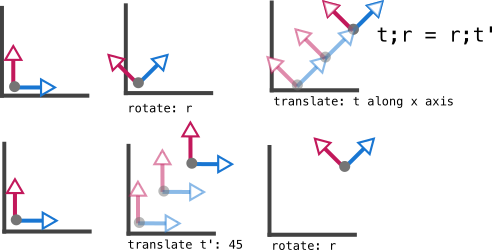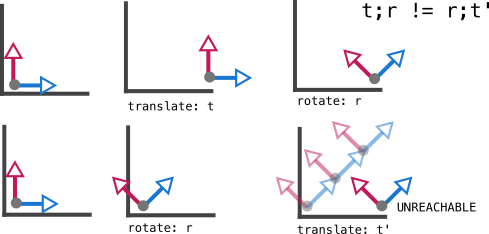## § Semidirect product as commuting conditions

Recall that in $N \ltimes K = G$, $N$ is normal. This is from the mnemonic that it looks like $N \triangleleft G$, or from the fact that the acting/twisting subgroup $K$ is a fish that wants to "eat"/act on the normal subgroup $N$. So, we have $knk^{-1} \in N$ as $N$ is normal, thus $knk^{-1} = n'$. This can be written as $kn = n'k$. So:
• When commuting, the element that gets changed/twisted in the normal subgroup. This is because the normal subgroup has the requisite constraint on it to be twistable.
• The element that remains invariant is the actor.
In the case of translations and rotations, it's the translations that are normal. This can be seen either by noticing that they are abelian, and are thus normal, while rotations don't "look normal". Alternatively, one can try to consider translate-rotate versus rotate-translate.• First rotating by $r$ and then translating by $t$ along the x-axis has the same effect as first translating by $t'$ at 45 degrees to the x-axis, and then rotating by the same r.
• This begs the question, is there some other translation t'' and some other rotation r'' such that t''; r'' ( t'' first, r'' next) has the same effect as r;t ( r first, t next)?• First let's translate by $t$ along the x-axis and then rotating by $r$. Now let's think, if we wanted to rotate and then translate, what rotation would we start with? it would HAVE TO BE $r$, since there's no other way to get the axis in such an angle in the final state. But if we rotate by $r$, then NO translation can get us to the final state we want. So, it's impossible to find a rotation;translationpair that mimics our starting translation;rotation.